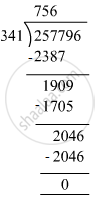# Divide and check your answer by the corresponding multiplication in the following: 257796 ​÷ 341 - Mathematics

Fill in the Blanks

Divide and check your answer by the corresponding multiplication in the following:

257796 ​÷ 341

#### SolutionDividend = 257796 , Divisor = 341 , Quotient = 756 , Remainder = 0
Check : Divisor × Quotient + Remainder = 341 × 756 + 0
= 257796
= Dividend
Hence, Dividend = Divisor × Quotient + Remainder

Is there an error in this question or solution?

#### APPEARS IN

RS Aggarwal Class 6 Mathematics
Chapter 3 Whole Numbers
Exercise 3E | Q 1.3 | Page 56